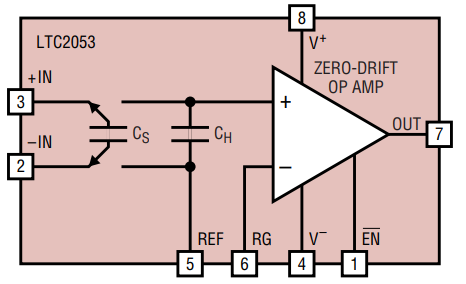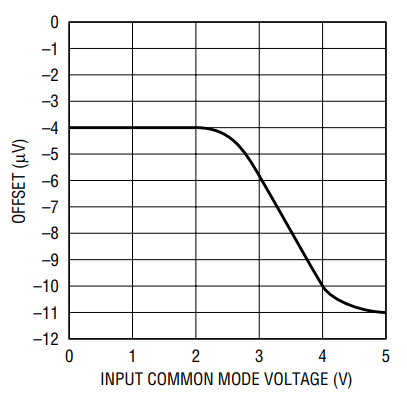# Unique Instrumentation Amplifier Precisely Senses Differential Voltages from µV to V

Introduction

The LTC2053 is the industry’s first instrumentation amplifier to feature a rail-to-rail input/output and a very high CMRR (guaranteed Common Mode Rejection Ratio of 110dB) that is gain-independent. This allows precision extraction of wide range of differential DC signals, from microvolts to volts, with common mode voltages anywhere between the supply rails.

The LTC2053 uses sophisticated charge balanced techniques to convert a differential input voltage into a single ended signal. Figure 1 shows the structure of the device in a simplified block diagram. A set of switches extract and store the input differential voltage across an internal sampling capacitor CS. This charge is then transferred into an internal hold capacitor, CH. With this operation, the differential input signal is extracted from the input common mode voltage and is referenced to the bias voltage of the REF pin. The signal is then further amplified by a zero-drift op amp connected in the non-inverting configuration. The negative input RG pin of the op amp is brought out to allow gain programmability and applications flexibility. Figure 2 shows how to program the gain (for gain greater than unity) using an external resistive divider (R1-R2)—where the gain is simply 1 + R2/R1. The tolerance of these resistors affects only the voltage gain accuracy of the circuit; not the CMRR. The LTC2053 is designed to work from 2.7V to 10V, single supply, or ±5V, dual supply. It is packaged in an MS8 surface mount package to save space, and consumes only 1mA of supply current when enabled, and less than 10µA when disabled by the EN pin.Figure 1. Block diagramFigure 2. Typical connection for gains higher than unity where: VOUT = VREF + (DVIN • Gain)

The Best DC Performance in The Industry

The LTC2053 uses auto-zeroing techniques to limit the maximum DC offset to only 10µV with a maximum DC offset drift of 50nV/°C. It also features a highly accurate 3ppm gain nonlinearity and 0.001% gain error— values unmatched by any other instrumentation amplifier available. The internal zero drift op amp of the LTC2053 does not significantly contribute to the overall DC error of the instrumentation amplifier, so the 110dB or more CMRR is gain-independent. This unique feature allows the LTC2053 to be used with low gains and still accommodate a huge differential signal dynamic range without losing DC precision—it can sense from several volts down to a few microvolts without requiring any gain adjustment. Figure 3 shows the DC offset of a typical device when both differential inputs are shorted together and then swept from rail to rail.Figure 3. DC offset vs common mode input for single 5V supply (gain = 1)

The LTC2053 Accommodates Rail-to-Rail Input Common Mode Voltages

The input common mode voltage range of the LTC2053 is from rail-to-rail—it can amplify DC differential signals riding on a common mode voltage of any voltage between the negative to the positive supply. The maximum allowable differential input voltage combined with the DC biasing of the reference pin is determined by the input common mode range (V to V+ – 1.3V) of the internal zero drift op amp. This can be simply expressed by:

Where V+IN and V–IN are the voltages of the input pins +IN and –IN respectively, and VREF is the voltage of the REF pin.

For single supply operation (V = 0) the maximum allowable differential input is from –VREF up to (V+ – 1.3V – VREF). The total differential input voltage range is therefore V+ – 1.3V.

For instance, if the LTC2053 is powered with a single 5V supply and if its reference pin is biased at +2.0V, the maximum differential input voltage for unity gain connection, V+IN – V–IN, can range from –2V to 1.7V. The total input differential voltage range is V+ – 1.3V = 3.7V.

With higher supply voltage operation, the voltage difference between either of the inputs, V+IN or V–IN, and the REF should be limited to 5.5V. For instance, for rail-to-rail input operation with ±5V supplies, the REF pin should be biased at 0V±0.5V. Or, if V+ is 10V and V and the REF pin are at 0V, the inputs should not exceed 5.5V.

Sensing Supply Currents

Figure 4 shows the LTC2053 sensing the load current of a voltage regulator across a 0.1mΩ shunt. Both inputs of the instrumentation amplifier are near the positive rail. The gain is 1001, and for a 5V supply and 5V full scale output, the LTC2053 output is rail-to-rail, the device can sense currents from 100mA (10µV input) all the way up to 50A (5mV input). In Figure 4, the 0.1µF across the 10k feedback resistor provides band limiting. The rail-to-rail input capability of the LTC2053 allows this circuit to be easily adapted to also sense ground currents.

3V Bridge Amplifier

Figure 5 illustrates the LTC2053 connected as a bridge amplifier. This straightforward circuit illustrates how easily this part can be applied. The supply voltage is a single 3V, the gain is 1001 and the DC common mode is half supply. Any AC common mode voltages up to 500Hz are rejected by 110dB.

Like all rail-to-rail op amps operating with a single supply, the LTC2053 output will not swing to zero volts when a zero input is presented; it will swing to within a few millivolts from ground. Therefore, the reference voltage can be tied to ground, as shown in Figure 5, provided the bridge is unidirectional and the circuit is not sensing a zero differential voltage—in which case the reference voltage would be biased at a voltage above ground to accommodate these conditions.

The impedance of the bridge should be equal or less than 10kΩ per leg. This ensures that any transient current that charges the parasitics of capacitor CS (which are not shown in the block diagram) has settled during the sampling phase of the LTC2053.

Using The RG Pin For More Than Setting Gain

Because the negative input of the internal op amp is brought out to the RG pin, active circuitry can be added inside the amplifier feedback loop without affecting the precision of the part. For instance, Figure 6 shows a concept for a circuit in which a discrete NPN transistor Q1 boosts the output current capability of the amplifier.